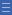## Featured resource

Home > Topdrawer > Mental computation > Activities > Facts and models

# Facts and models

Facts and models

In this game, students draw on their knowledge of fact families to generate multiplication and division facts.

Card and dice games provide a motivational way for students to develop recall of known multiplication and division facts.

### Facts and models game

Materials: Deck of playing cards (picture cards = 0, Ace = 1), tiles or counters, blank paper.

• The aim of the game is to be the first to use four cards to make a multiplication or division fact.
• Play in pairs. Each player draws five cards from the deck and uses four of them to make a multiplication or division fact.
For example, Player A draws 4, 8, 3, 2, 6 and can make 32 ÷ 4 = 8 or 8 $$\times$$ 4 = 32.
• A point is scored for each multiplication or division fact generated. An additional point is scored for explaining thinking (e.g. "I know 8 twos are 16 and double it is 8 fours.")
• The partner may suggest other facts, and can check by making a pictorial representation or concrete model using tiles or counters.
• If unable to make a multiplication or division fact with the four cards, a player can opt to discard a card and take another from the deck. This is considered a turn.
• If a 10 is drawn it can be put with another card and read as a 3-digit number (e.g. 106). The player can choose to discard the 10 and take another card from the deck.
• The game ends when the deck is exhausted.

You can watch the Facts and Models video.

Players list the different facts generated for each product in a table and discuss any connections between them.

 12 20 27 32 4 $$\times$$ 3 = 12 4 $$\times$$ 5 = 20 3 $$\times$$ 9 = 27 8 $$\times$$ 4 = 32 3 $$\times$$ 4 = 12 5 $$\times$$ 4 = 20 9 $$\times$$ 3 = 27 4 $$\times$$ 8 = 32 12 ÷ 4 = 3 20 ÷ 4 = 5 27 ÷ 3 = 9 32 ÷ 8 = 4 12 ÷ 3 = 4 20 ÷ 5 = 4 29 ÷ 9 = 3 32 ÷ 4 = 8

As a class, discuss any facts that were difficult to calculate and strategies that might assist other students.

Yes

Yes

Name Class SectionYear 4: Recall multiplication facts up to 10 x 10 and related division facts Infobox 3Year 3: Recall multiplication facts of two, three, five and ten and related division facts Infobox 3Source Infobox 3